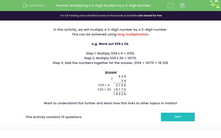# Multiplying a 3-Digit Number by a 2-Digit Number

In this worksheet, students multiply a 3-digit number by a 2-digit number.Key stage:  KS 2

Curriculum topic:   Number: Multiplication and Division

Curriculum subtopic:   Multiply Numbers to Four Digits

Difficulty level:#### Worksheet Overview

In this activity, we will multiply a 3-digit number by a 2-digit number.

This can be achieved using long multiplication.

e.g. Work out 539 x 34.

Step 1: Multiply 539 x 4 = 2156.

Step 2: Multiply 539 x 30 = 16170.

Step 3: Add the numbers together for the answer. 2156 + 16170 = 18 326

 × 5 3 9 3 4 539 × 4 2 1 5 6 539 × 30 1 6 1 7 0 1 8 3 2 6

Want to understand this further and learn how this links to other topics in maths?

### What is EdPlace?

We're your National Curriculum aligned online education content provider helping each child succeed in English, maths and science from year 1 to GCSE. With an EdPlace account you’ll be able to track and measure progress, helping each child achieve their best. We build confidence and attainment by personalising each child’s learning at a level that suits them.

Get started